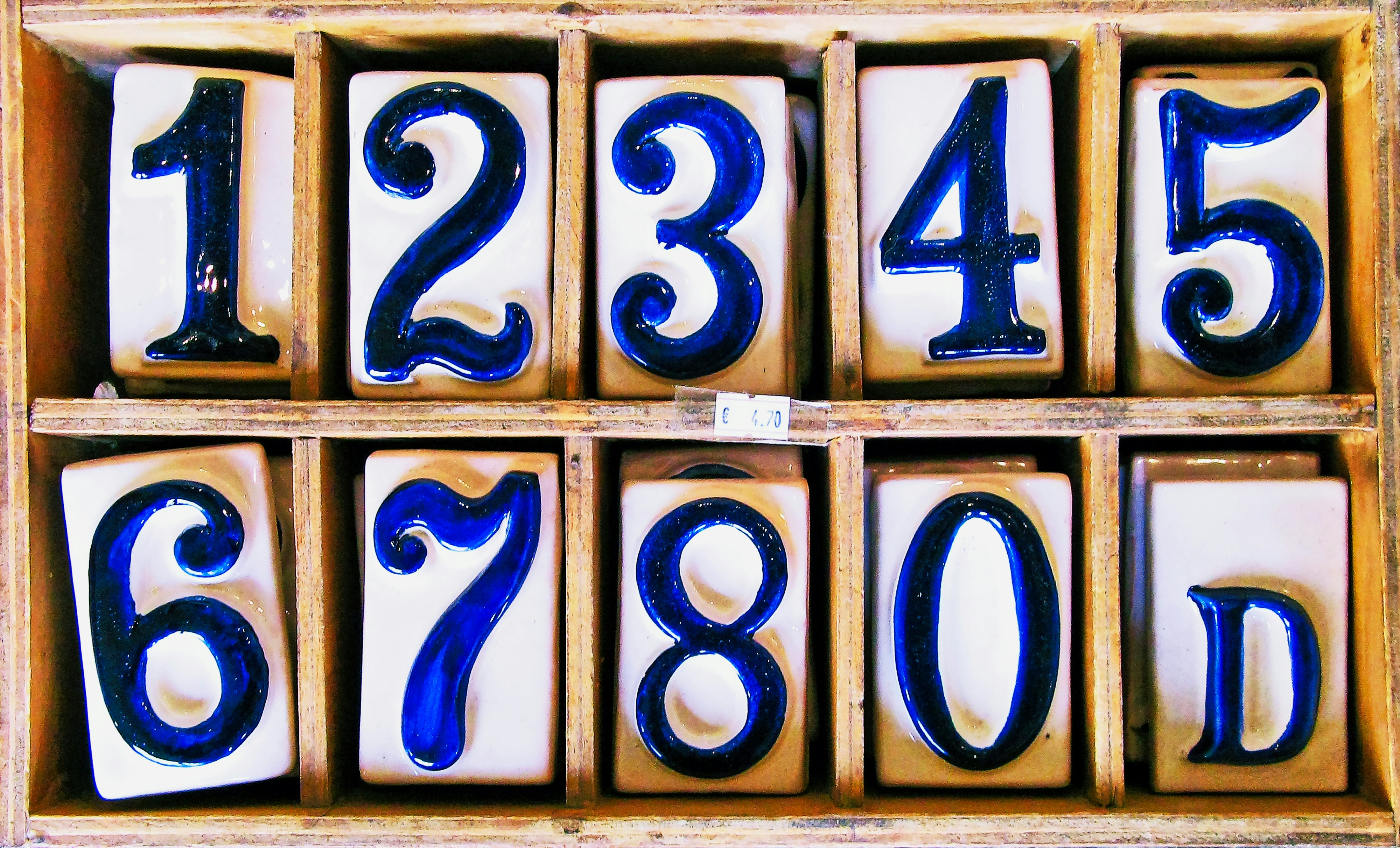#DAY 27
0
Modern Web

## NaN, not a number

2020 秋天，我將用 30 天的時間，來嘗試回答和網路前端開發相關的 30 個問題。30 天無法一網打盡浩瀚的前端知識，有些問題可能對有些讀者來說相對簡單，不過期待這趟旅程，能幫助自己、也幫助讀者打開不同的知識大門。有興趣的話，跟著我一起探索吧！## What is NaN?

Number value that is an IEEE 754-2019 “Not-a-Number” value

In computing, NaN, standing for Not a Number, is a member of a numeric data type that can be interpreted as a value that is undefined or unrepresentable, especially in floating-point arithmetic. Systematic use of NaNs was introduced by the IEEE 754 floating-point standard in 1985, along with the representation of other non-finite quantities such as infinities.

## What will generate NaN?

• `0 / 0` (基本上這在數學中是無意義的運算式)
• `Infinity or -Infinity / Infinity or -Infinity`
• `Infinity``-Infinity` 與 0 相乘
• `x % y`，而 x 是無限大，y 等於 0
• `Infinity`, `-Infinity` 彼此（或自己）進行加減運算
• ...

## Comparison with NaN

``````NaN > 1         // false
NaN < 1         // false
NaN == 1        // false
NaN === 1       // false
``````

``````NaN == NaN       // false
NaN === NaN      // false
``````

``````NaN != 1         //  true
NaN !== 1        //  true
NaN != NaN       //  true
NaN !== NaN      //  true
``````

## NaN in JavaScript

``````typeof NaN        // number
``````

``````NaN + 1           // NaN
NaN - 1           // NaN
NaN * 1           // NaN
NaN / 1           // NaN
``````

``````NaN >> 1          // 0
NaN << 1          // 0
NaN ^ 0           // 0
``````

``````Number(NaN)       // NaN
String(NaN)       // "NaN"
``````

``````isNaN(0)           // false
isNaN(1)           // false
isNaN(Infinity)    // false
isNaN(NaN)         // true
isNaN()            // true
isNaN([1,2,3])     // true
isNaN({age: 18})   // true
``````

• Infinity 本身還是個 number
• NaN 雖然是 numeric data type，但卻是 not a number

## NaN in array

``````let arr = [2, 4, NaN, 12];
arr.indexOf(NaN);                      // -1 (false)
arr.includes(NaN);                     // true
arr.findIndex(n => Number.isNaN(n));   // 2
``````

## End

• 數學無法計算出來的東西，就會用 NaN 代表
• NaN 本身的型別是 number，但它沒有一個固定的數值，它是 not a number
• 沒辦法用 indexOf 找到 array 當中的 NaN

## Ref

TD
Be curious as astronomer, think as physicist, hack as engineer, fight as baseball player